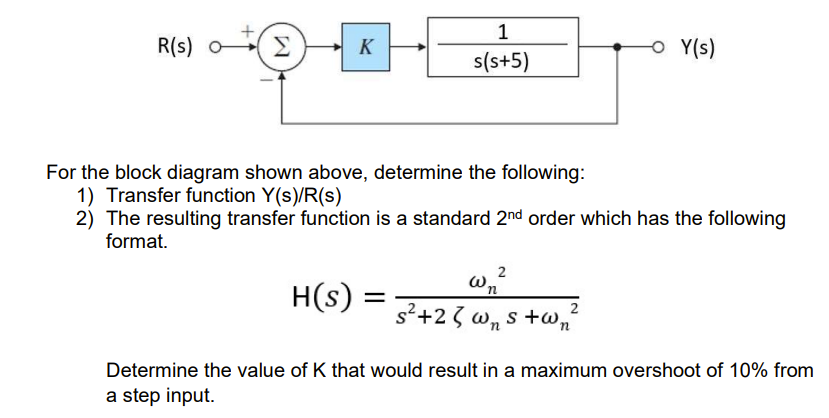# Question For the block diagram shown above, determine the following: 1) Transfer function $$Y(s) / R(s)$$ 2) The resulting transfer function is a standard $$2^{\text {nd }}$$ order which has the following format. $H(s)=\frac{\omega_{n}^{2}}{s^{2}+2 \zeta \omega_{n} s+\omega_{n}^{2}}$ Determine the value of $$\mathrm{K}$$ that would result in a maximum overshoot of $$10 \%$$ from a step input.8IUY43 The Asker · Mechanical EngineeringTranscribed Image Text: For the block diagram shown above, determine the following: 1) Transfer function $$Y(s) / R(s)$$ 2) The resulting transfer function is a standard $$2^{\text {nd }}$$ order which has the following format. $H(s)=\frac{\omega_{n}^{2}}{s^{2}+2 \zeta \omega_{n} s+\omega_{n}^{2}}$ Determine the value of $$\mathrm{K}$$ that would result in a maximum overshoot of $$10 \%$$ from a step input.
More
Transcribed Image Text: For the block diagram shown above, determine the following: 1) Transfer function $$Y(s) / R(s)$$ 2) The resulting transfer function is a standard $$2^{\text {nd }}$$ order which has the following format. $H(s)=\frac{\omega_{n}^{2}}{s^{2}+2 \zeta \omega_{n} s+\omega_{n}^{2}}$ Determine the value of $$\mathrm{K}$$ that would result in a maximum overshoot of $$10 \%$$ from a step input.